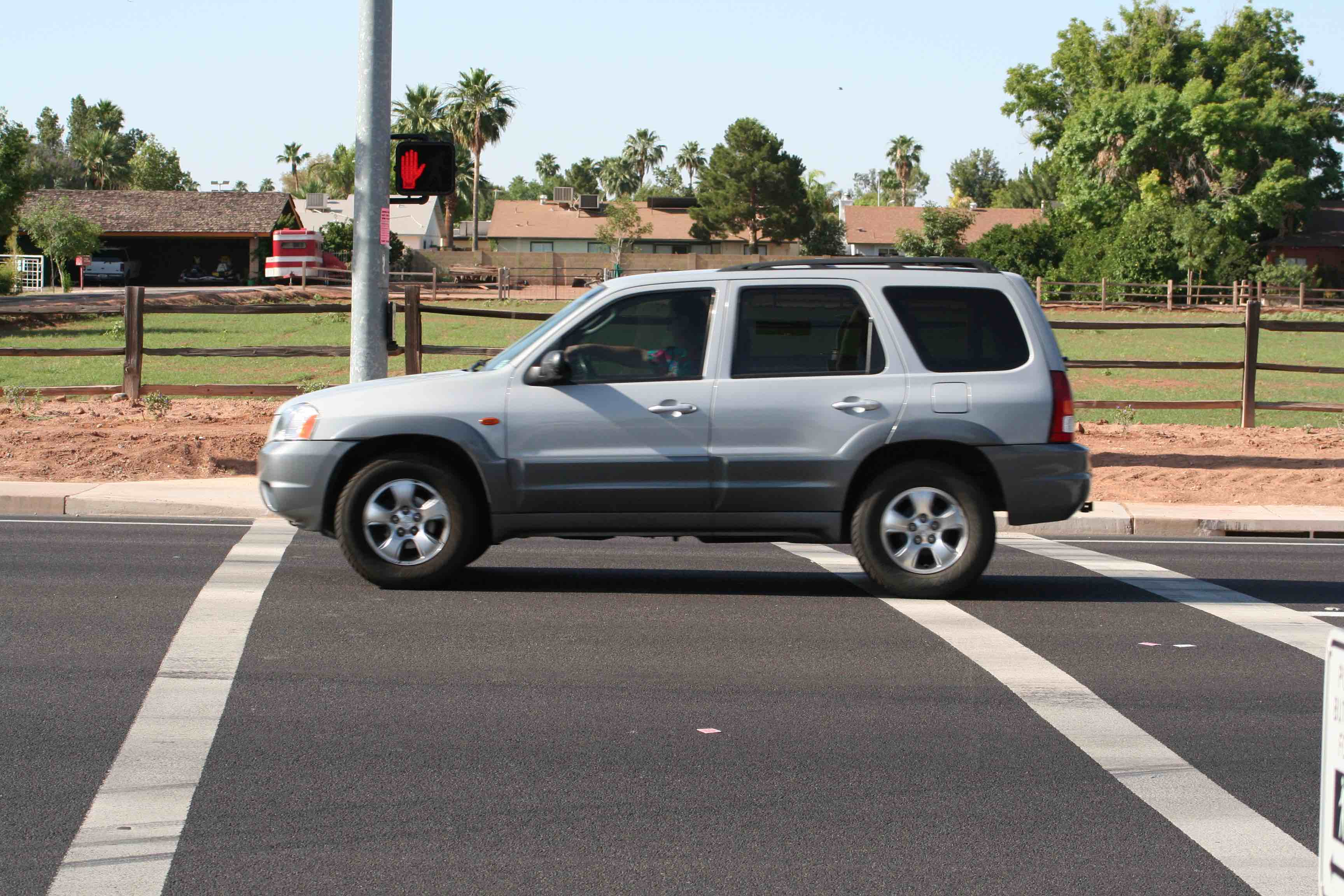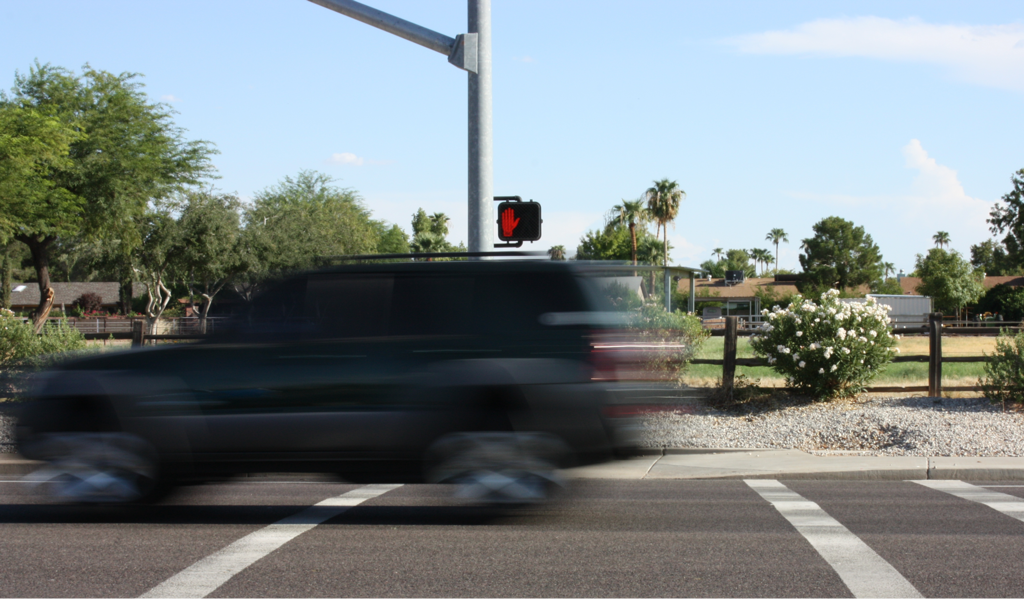< Previous Section Home Next Section >

# Section 4.3Differentials in Action

Suppose a car accelerates. What do you understand that we mean when we say, "At exactly 2.25 seconds after starting, the car is going exactly 64.8 km/hr"?

• Does it mean the car’s speedometer is pointing at exactly 64.8 exactly 2.25 sec after starting? It might, but the car could be traveling at exactly 64.8 km/hr even with a broken or inaccurate speedometer.
• Does it mean the car’s number of km traveled is 64.8 times the number of hours it traveled? It cannot. It accelerated up until that moment, and at that exact moment the car hasn’t moved!

There are several meanings that we can give to "… going exactly 64.8 km/hr". One is that, for a very small interval of time around 2.25 seconds, the car moved essentially at a constant rate of 64.8 km/hr. For that brief period of time we are unable, by any means, to discern a variation in its speed.

Figure 4.3.1 shows a photo of a SUV at a stop light taken with the shutter open for 1/1000 sec. Is it stopped or moving? It appears that the SUV is stopped.Figure 4.3.1. A photo of a SUV taken with a shutter setting of $\frac{1}{1000}$ sec. Is it stopped or moving?

When we zoom in on the SUV’s midsection (Figure 4.3.2), we see tiny streaks in the photo. The streaks were made by the SUV having moved slightly during the 1/1000 sec that the shutter was open.

Figure 4.3.2. A closer look at the SUV’s midsection shows small streaks. The SUV moved slightly while the
camera's shutter was open. A moment in time is a small interval of time.

Let D represent the number of meters that the SUV moved that day, let t represent the number of seconds during which the SUV traveled D meters, and let s represent the SUV’s speed (in m/s) at the moment the shutter opened.

For the 1/1000 sec that the shutter was open, and assuming the SUV’s speed was essentially constant during that time, we can say two things:

• for $0\lt dt \le 0.001$ sec, $dD=s\cdot dt$ meters, so
• when $dt=0.001$ sec, $dD=s\cdot 0.001$ meters.

In words, we would say that

• the variation in the SUV’s number of meters driven ($dD$)
• is its speed in m/sec (s)
• times the number of seconds ($dt$) that the shutter has been open.

Reflection 4.3.1. What is the difference in the meanings between saying, "The SUV’s speed was essentially constant during that time" and saying, "The SUV’s speed was constant during that time"?

Reflection 4.3.2. Suppose D represents the number of meters that the SUV had traveled since it was manufactured instead of the number of meters it traveled that day. How would you need to adjust the statement $dD$ = s∙0.001 meters so that it would describe the SUV's variation in distance traveled while the camera's shutter was open? Why?

## Exercise Set 4.3

Exercises 1-3 refer to this photograph.1. The photo above was taken with the camera shutter open for 1/20 sec. The distance between white stripes is 3 meters. The length of the blur is the distance the car traveled while the shutter was open.
1. Approximately how fast, in km/hr, was the car traveling?
2. Study the video at this link after you finish part (a). Compare your reasoning in 1.a with the reasoning portrayed in the video. Re-work part (a) if you realize an error in your thinking.
3. What is your interpretation of the video’s moral, "all motion is blurry"? How are differentials of distance traveled and time traveled related to this moral?
2. Express the variation in the car’s distance traveled in terms of differentials of numbers of km it traveled and numbers of hours it traveled.
3. Sketch a graph of the car’s distance traveled (in km) with respect to the time it traveled (in hours) during the time this photo was formed, given that the car had traveled 35.7 km up to the moment this photo was taken. How would the graph change had the car traveled 146.2 km up to the moment this photo was taken?
4. Employ the same reasoning as illustrated in Exercise 1 to estimate the speeds of these vehicles traveling through the same crosswalk.
1. Photo taken at 1/30th second exposure.
2. Photo taken at 1/60th second exposure.
3. Photo taken at 1/100th second exposure.
5. Approximately how many meters did the automobile in 4.a travel in 1/90 sec? Use differentials to express the variation in distance with respect to the variation in time during this 1/90 sec.
6. Approximately how many meters did the automobile in 4.b travel in 1/45 sec? Use differentials to express the variation in distance with respect to the variation in time during this 1/45 sec.
7. Approximately how many meters did the automobile in 4.c travel in 1.7 sec? Use differentials to express the variation in distance with respect to the variation in time during this 1.7 sec.
8. A toy car, traveling at a constant speed of 5 ft/sec, travels 13 feet.
1. The track is marked with intervals of length 1 ft. How many seconds does the car spend in each interval?
2. The track is marked with intervals of length 1/250 ft. How many seconds does the car spend in each interval?
3. The track is marked with intervals of length 1/5000 ft. How many seconds does the car spend in each interval?
4. The track is marked with intervals of length $\Delta d$ ft ($\Delta d$ can be a tiny, tiny number). How many seconds does the car spend in each interval?
5. What do questions 8a-8d have to do with differentials?

 < Previous Section Home Next Section >